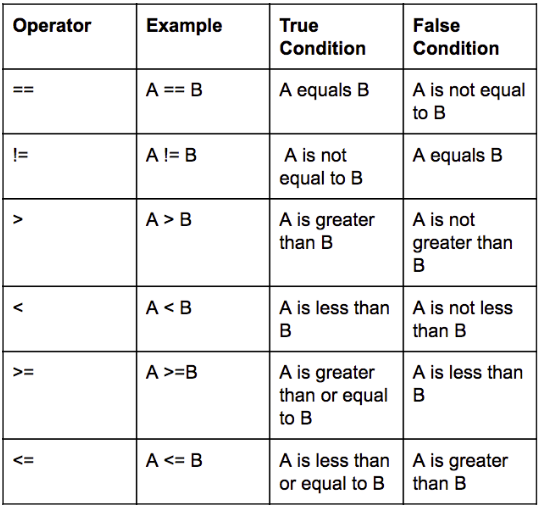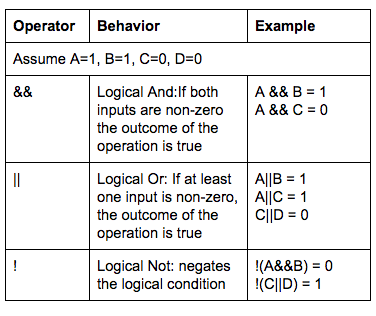C++的控制流，非常简单。

# 1. Intro to Control Flow

C++ has several control flow options.

• if-else statements
• for loops
• while loops
• switch statements

# 2. Relational Operators``````/*Goal: learn if-else statements in C++*/

#include<iostream>
#include<string>

int main()
{
//instead of printing 0 and 1, create an array where
//0 = False, 1 = True
std::string TorF[] = {"False", "True"};

int a = 100;
int b = 33;
int c = 33;

//Print out the string values of each relational operation
std::cout<<"a < b is "<<TorF[a<b];
std::cout<<"\na > b is "<<TorF[a>b];
std::cout<<"\na != b is "<<TorF[a!=b];
std::cout<<"\nc >= b is "<<TorF[c>=b];
std::cout<<"\nc <= b is "<<TorF[c<=b];
return 0;
}
``````
``````a < b is False
a > b is True
a != b is True
c >= b is True
c <= b is True
``````

# 3. Logic Operators``````/*Goal: understand the logical operators supported by C++
*/

#include<iostream>
#include<string>

int main()
{
int A = 5;
int B = 4;
int C = 5;
int D = 0;

std::string TorF[] = {"False", "True"};

//The && operator
std::cout<<"A == C is "<<TorF[A==C];
std::cout<<"\n(B == D) is "<<TorF[B==D];
std::cout<<"\n(B > D) is "<<TorF[B>D];
//A true && false = false
std::cout<<"\n\n(A ==C) && (B == D) is "<<TorF[(A ==C) && (B == D)];
//A true and true = true
std::cout<<"\n(A ==C) && (B > D) is "<<TorF[(A ==C) && (B > D)];

//The || operator
//A true || false = true
std::cout<<"\n\n(A ==C) || (B == D) is "<<TorF[(A ==C) || (B == D)];
//A true || true = true
std::cout<<"\n(A ==C) || (B > D) is "<<TorF[(A ==C) || (B > D)];

//The 'Not' operator
std::cout<<"\n\nA < B is "<<TorF[A<B];
std::cout<<"\n!(A < B) is "<<TorF[!(A<B)];

std::cout<<"\n\nA == C is "<<TorF[A==C];
std::cout<<"\n!(A == C) is "<<TorF[!(A==C)];

return 0;
}
``````
``````A == C is True
(B == D) is False
(B > D) is True

(A ==C) && (B == D) is False
(A ==C) && (B > D) is True

(A ==C) || (B == D) is True
(A ==C) || (B > D) is True

A < B is False
!(A < B) is True

A == C is True
!(A == C) is False
``````

# 8. if statements

``````/*Goal: use if statements*/

#include<iostream>

int main()
{
int a = 10;

std::cout<<"This program checks the value of a.\n";

if(a == 6)
{
std::cout<<"a is equal to a half dozen.\n";
}

std::cout<<"There is not much to say about a\n";
return 0;
}
``````

# 9. if-else Statements

``````/*Goal: learn if-else statements in C++
**The user guess in in the input.txt file
**Change the value to test each of the possible
**code branches.*/

#include<iostream>

int main()
{
int TARGET = 33;
int guess;
std::cout<<"Guess a number between 0 - 100\n";
std::cin>>guess;

std::cout<<"You guessed: "<<guess<<"\n";

if(guess < TARGET)
{
}
else if(guess > TARGET)
{
}
else
{
std::cout<<"Yay! You guessed correctly.\n";
}

return 0;
}
``````
``````/*Goal: practice if-else statements in C++
**Write a program to select the best pet.
*/

#include<iostream>

int main()
{
char skin, location;
std::cout<<"Would you like an animal with fur(f), feathers(t), or scales(s)?";
std::cin>>skin;
std::cout<<skin<<"\n";

//Use if-else statements to control program flow
if(skin == 'f')
{
std::cout<<"Get a dog"<<"\n";
}
else if(skin == 't')
{
std::cout<<"Get a bird"<<"\n";
}
else if(skin == 's')
{
std::cout<<"Would you like an animal that lives in water(w), land(l), or both(b)?";
std::cin>>location;
std::cout<<location<<"\n";

//Use if-else statements to control program flow
//note that the curly braces are not necessary if there is only
//one line of code.

if(location == 'w')
std::cout<<"Get a fish"<<"\n";
else if(location == 'l')
std::cout<<"Get a gecko"<<"\n";
else if(location == 'b')
std::cout<<"Get a frog"<<"\n";
else
std::cout<<"Enter water(w), land(l), or both(b)\n";
}
else
return 0;
}
``````

# 14. switch statements

``````/*Goal: demonstrate use cases for the switch statement.*/

#include <iostream>

int main()
{

std::cout<<"What is your favorite winter sport?: \n";
std::cout<<"1.Skiing\n2: Sledding\n3: Sitting by the fire";
std::cout<<"\n4.Drinking hot chocolate\n";
std::cout<<"\n\n";

{
case(1): std::cout<<"Skiing?! Sounds dangerous!\n";
break;
case(2): std::cout<<"Sledding?! Sounds like work!\n";
break;
case(3): std::cout<<"Sitting by the fire?! Sounds warm!\n";
break;
case(4): std::cout<<"Hot chocolate?! Yum!\n";
break;
default: std::cout<<"Enter a valid menu item";
}

char begin;
std::cout<<"\n\nWhere do you want to begin?\n";
std::cout<<"B. At the beginning?\nM. At the middle?";
std::cout<<"\nE. At the end?\n\n";
begin = 'M';

switch(begin)
{
case('B'): std::cout<<"Once upon a time there was a wolf.\n";
case('M'): std::cout<<"The wolf hurt his leg.\n";
case('E'): std::cout<<"The wolf lived happily everafter\n";
}
return 0;
}
``````
``````What is your favorite winter sport?:
1.Skiing
2: Sledding
3: Sitting by the fire
4.Drinking hot chocolate

Skiing?! Sounds dangerous!

Where do you want to begin?
B. At the beginning?
M. At the middle?
E. At the end?

The wolf hurt his leg.
The wolf lived happily everafter
``````

``````/*Goal: understand the switch statement in C++
**This example does not use a break statement between
**the possibilities, which means all menu items below the selected
**one are executed.
*/

#include<iostream>

int main()
{
std::cout<<"L: luxury package\nS: standard package\n";
std::cout<<"B: basic package ";

{
case 'L':
{
std::cout<<"\tSpa Day\n";
std::cout<<"\tSailboat Tour\n";
}
case 'S':
{
std::cout<<"\tCity Tour\n";
std::cout<<"\tComplimentary Happy Hour\n";
}
case 'B':
{
std::cout<<"\tAirport Transfers\n";
std::cout<<"\tComplimentary Breakfast\n";
break;
}
default:
}
return 0;
}

``````

``````Choose your holiday package:
L: luxury package
S: standard package
B: basic package L
The L package includes:
Spa Day
Sailboat Tour
City Tour
Complimentary Happy Hour
Airport Transfers
Complimentary Breakfast
``````

# 14. switch Programming quiz

``````/*Now I would like you to do a switch statement with breaks
**between the cases. Create a program that asks the user for
**two float numbers. Then asks the user if they would like to:
**add the numbers, subtract the numbers, multiply the numbers,
**divide the numbers.
**The program should then print the numbers with the chosen
**operation and the solution.
*/

#include <iostream>

int main()
{
float in1, in2;
char operation;

std::cout<<"Enter two numbers:\n";
std::cin>>in1;
std::cin>>in2;
std::cout<<"Enter the operation '+','-','*','/':\n";
std::cin>>operation;

switch(operation)
{
case('+'):  {
break;
}
case('-'):  {
break;
}
case('*'):  {
break;
}
case('/'):  {
break;
}
default:
std::cout<<"Illegal operation";
}

return 0;
}
``````

# 16. for Loops

``````/*Write a program that asks a user for five numbers.
**Print out the sum and average of the five numbers.
*/

#include <iostream>

int main()
{
float input;
float sum = 0;

for(int i=0;i<5;i++)
{
std::cout<<"What is the next number?\n";
std::cin>>input;
sum = sum + input;
}//end of for loop

std::cout<<"Sum = "<<sum<<"\n";
std::cout<<"Average = "<<sum/5<<"\n";
return 0;
}

``````

# 19. while Loops

``````/*Goal: understand the syntax of while loops*/

#include <iostream>

int main()
{
int entry = 0;

//with this while loop the condition is true
//so the statements are executed
while(entry <=5)
{
std::cout<<"incrementing entry = "<<entry<<"\n";
entry++;
}

std::cout << "--------------------------------" << "\n";
//with this while loop the condition is false
//so the statements are not executed
while((entry < 10) &&(entry > 0))
{
std::cout<<"decrementing entry = "<<entry<<"\n";
entry--;
}

return 0;
}
``````
``````incrementing entry = 0
incrementing entry = 1
incrementing entry = 2
incrementing entry = 3
incrementing entry = 4
incrementing entry = 5
--------------------------------
decrementing entry = 6
decrementing entry = 5
decrementing entry = 4
decrementing entry = 3
decrementing entry = 2
decrementing entry = 1
``````

# 20. do while Loops

The statements are executed the first time through the loop. Then the condition is checked. This is slightly different than the while loop. Which, you may recall, required the condition to be true BEFORE entering the loop for the first time.

``````/*Goal: understand the do..while loop*/

#include <iostream>

int main()
{
int count = 0;

//This do..while loop will execute until count =5
do
{
std::cout<<"Count = "<<count<<"\n";
count++;
}while(count < 5);

int otherCount = 6;
//This do..while loop will execute once. Even though
//otherCount > 5
do
{
std::cout<<"othercount = "<<otherCount<<"\n";
otherCount++;
}while(otherCount < 5);

return 0;
}

``````
``````Count = 0
Count = 1
Count = 2
Count = 3
Count = 4
othercount = 6
``````

# 21. while Loops Programming Quiz

``````/*Goal: In the programming quiz, use a while loop to prompt
**the user to guess a target number.
**Tell the user if the guess is too high or too low.
**The user enters -1  or guesses the target number to end
**the program.
*/
#include <iostream>
#include<sstream>

int main()
{
int target = 55;
std::string userString;
int guess = -1;

while(guess != target )
{
std::cout<<"Guess a number between 0 and 100: ";
std::cin>>guess;
std::cout<<guess<<"\n";
if(guess > target)
else if(guess < target)
else
std::cout<<"You guessed the target!\n";

//Note I had to use double quotes around "q"
//because it is a string
if(guess == -1)
{
std::cout<<"good bye!";
break;
}
}
return 0;
}
``````
``````/*Goal: In the programming quiz, use a while loop to prompt
**the user to guess a target number.
**Tell the user if the guess is too high or too low.
**The user enters 'q'  or guesses the target number to end
**the program.
*/

#include <iostream>
#include<sstream>
#include <time.h> //added for the random number generator seed
#include <cstdlib>//added to use the rand function

int main()
{
int target;
std::string userString;
int guess = -1;

srand(time(NULL));  //set the seed for the random number generator
target = rand() %100 + 1; //generate the 'random' number

while(guess != target)
{
std::cout<<"Guess a number between 0 and 100: ";
std::getline (std::cin,userString);
//convert to an int
std::stringstream(userString) >> guess;
std::cout<<userString<<"\n";
if(guess > target)
else if(guess < target)
else
std::cout<<"You guessed the target!\n";

//Note I had to use double quotes around "q"
//because it is a string
if(userString == "q")
{
std::cout<<"good bye!";
std::cout<<"The number was "<<target<<"\n";
break;
}
}
return 0;
}

``````

# 24. Infinite Loops

Sometimes it is desired to create an infinite loop. For example, in embedded systems a infinite loop is often used for the main task.

``````for( ; ;)
{
std::cout<<"This for loop will run forever\n";
}
``````
``````while(1)
{
std::cout<<"This while loop will run forever\n";
}
``````

# 25. Exiting Loops

• break

The break statement will end the loop and begin executing the first statement that comes AFTER the end of the loop.

• continue

The continue statement will force the next iteration to be executed.

``````/*Goal: understand the break and conitnue statements*/

#include<iostream>

int main()
{
int a = 0;
while(a < 6)
{
std::cout<<"a = "<<a<<"\n";
a++;
if(a == 3)
break;
}
std::cout<<"The first statement after the first while loop\n\n";

while(a < 12)
{
a++;
if(a == 10)
{
std::cout<<"\tWhen a=10, go back to the top of the loop";
std::cout<<"\n\tThis means a=10 is skipped.\n";
continue;
}
std::cout<<"After continue a = "<<a<<"\n";
}
return 0;
}
``````
``````a = 0
a = 1
a = 2
The first statement after the first while loop

After continue a = 4
After continue a = 5
After continue a = 6
After continue a = 7
After continue a = 8
After continue a = 9
When a=10, go back to the top of the loop
This means a=10 is skipped.
After continue a = 11
After continue a = 12

``````